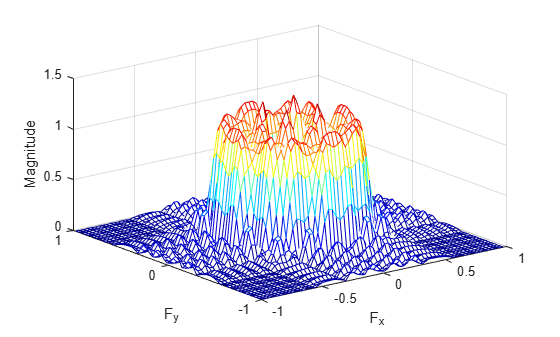# fsamp2

2-D FIR filter using frequency sampling

## Syntax

``h = fsamp2(Hd)``
``h = fsamp2(f1,f2,Hd,[m n])``

## Description

example

````h = fsamp2(Hd)` designs a two-dimensional FIR filter with frequency response `Hd`, and returns the filter coefficients in matrix `h`. The filter `h` has a frequency response that passes through points in `Hd`. `fsamp2` designs two-dimensional FIR filters based on a desired two-dimensional frequency response sampled at points on the Cartesian plane. ```
````h = fsamp2(f1,f2,Hd,[m n])` produces an `m`-by-`n` FIR filter by matching the filter response at the points in the vectors `f1` and `f2`. The frequency vectors `f1` and `f2` are in normalized frequency, where 1.0 corresponds to half the sampling frequency, or π radians. The resulting filter fits the desired response as closely as possible in the least squares sense. For best results, there must be at least `m*n` desired frequency points. `fsamp2` issues a warning if you specify fewer than `m*n` points.```

## Examples

collapse all

Use `fsamp2` to design an approximately symmetric, two-dimensional bandpass filter with passband between 0.1 and 0.5 (normalized frequency, where 1.0 corresponds to half the sampling frequency, or $\pi$ radians).

Create a matrix `Hd` that contains the desired bandpass response. Use `freqspace` to create the frequency vectors `f1` and `f2`.

```[f1,f2] = freqspace(21,'meshgrid'); Hd = ones(21); r = sqrt(f1.^2 + f2.^2); Hd((r<0.1)|(r>0.5)) = 0; colormap(jet(64)) mesh(f1,f2,Hd)```Design the filter that passes through this response.

```h = fsamp2(Hd); freqz2(h)```## Input Arguments

collapse all

Frequency response, specified as a numeric matrix. `Hd` is a matrix containing the desired frequency response sampled at equally spaced points between -1.0 and 1.0 along the x and y frequency axes. The value 1.0 corresponds to half the sampling frequency, or π radians.

`${H}_{d}\left({f}_{1},{f}_{2}\right)={{H}_{d}\left({\omega }_{1},{\omega }_{2}\right)|}_{{\omega }_{1}=\pi {f}_{1},{\omega }_{2}=\pi {f}_{1}}$`

For best results, use frequency points returned by `freqspace` to create `Hd`.

Data Types: `single` | `double` | `int8` | `int16` | `int32` | `int64` | `uint8` | `uint16` | `uint32` | `uint64`

Frequency vector, specified as a numeric vector.

Data Types: `double`

Frequency vector, specified as a numeric vector.

Data Types: `double`

Size of output FIR filter `h`, specified as a 2-element vector of positive integers. The filter has `m` rows and `n` columns.

Data Types: `double`

## Output Arguments

collapse all

2-D FIR filter, returned as a numeric array. `fsamp2` returns `h` as a computational molecule, which is the appropriate form to use with `filter2`. If you specify a frequency response matrix `Hd`, then `h` has the same size. If `Hd` is of class `single`, `h` is also of class `single`. Otherwise, `h` is of class `double`.

Data Types: `single` | `double`

## Algorithms

`fsamp2` computes the filter `h` by taking the inverse discrete Fourier transform of the desired frequency response. If the desired frequency response is real and symmetric (zero phase), the resulting filter is also zero phase.

 Lim, Jae S., Two-Dimensional Signal and Image Processing, Englewood Cliffs, NJ, Prentice Hall, 1990, pp. 213-217.